### Home > INT2 > Chapter 12 > Lesson 12.2.1 > Problem12-69

12-69.
1. Carefully examine each diagram below and explain why the geometric figure cannot exist. Support your statements with reasons. If a line looks straight, assume that it is. Homework Help ✎

2.  a.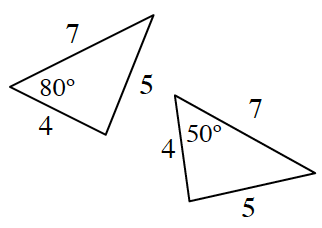b.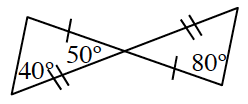c.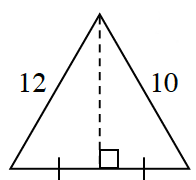d.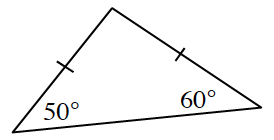e.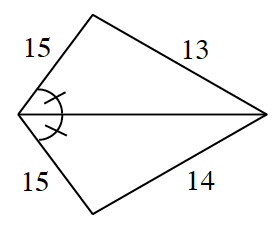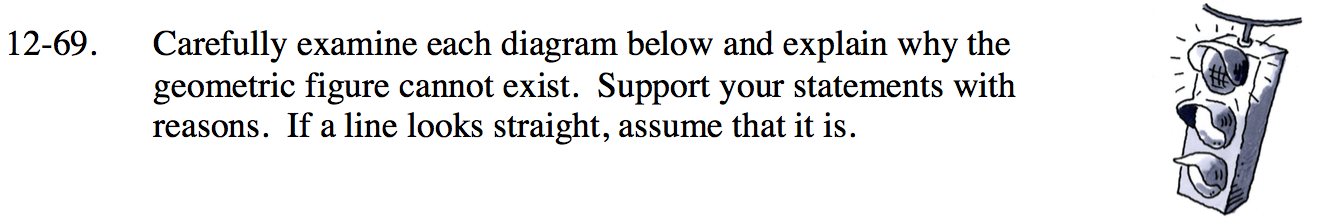Review the triangle congruence conjectures from the Math Notes box.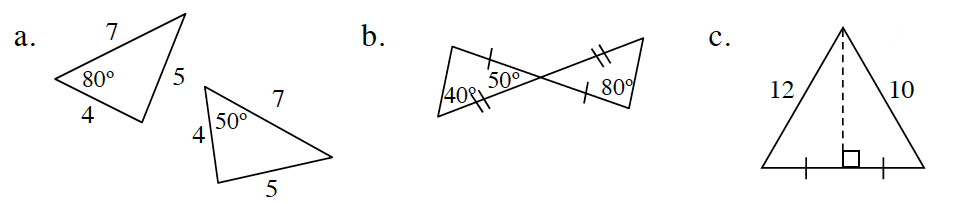The triangles should be congruent by SSS ≅, but 80° ≠ 50° and in congruent triangles all angles are equal as well.

The triangles should be congruent by SAS ≅, but 80° ≠ 90° and 40° ≠ 50° and in congruent triangles all angles are equal as well.

The triangles should be congruent by SAS ≅, but 10 ≠ 12 and in congruent triangles all sides are congruent as well.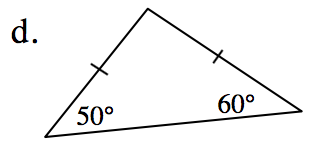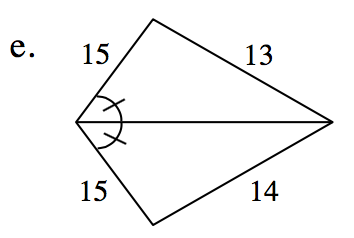What do you know about isosceles triangles?